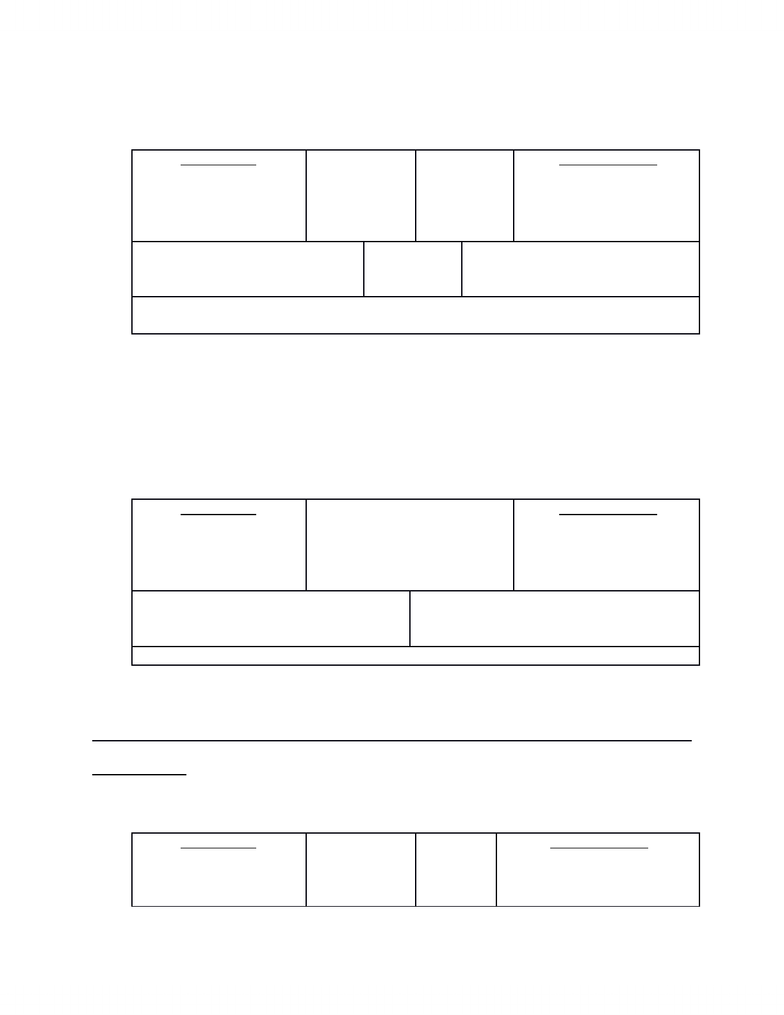Class Notes (1,100,000)
CA (630,000)
Ryerson (30,000)
ACC 406 (200)
Lecture

# Standard Costing

Department
Accounting
Course Code
ACC 406
Professor
Alison Beavis

This preview shows half of the first page. to view the full 3 pages of the document.Chapter 10 & 11: Variance Analysis
1)Variance Analysis for Direct Materials:
Actual Cost
Actual Quantity (10)
X
Actual Price/unit (\$2)
AQpurchased (10)
X
SPpurchased
(\$1.5)
AQused
X
SPused
Standard Cost
(Expectations)
Standard Quantity (12)
X
Standard Price/unit (\$1.5)
Direct Materials Price Variance
= Difference
\$5 (Unfavorable)
Direct Materials Usage Variance
= Difference
\$3 (Favorable)
Total Direct Materials Variance = Difference
\$2 (Unfavorable)
Increasing Unfavorable Increasing
Favorable
Direct Materials Framework:
Standard Quantity = Actual Output * Input-Output Ratio
2)Variance Analysis for Direct Labor:
Actual Cost
Actual Hours
X
Actual Rate/hour
AH
X
SR
Standard Cost
(Expectations)
Standard Hours
X
Standard Rate/hour
Direct Labor Rate Variance = Difference
(AH) (SR AR)Direct Materials Efficiency Variance =
Dfference
(SR) (SH AH)
Total Direct Labor Variance = Difference
Increasing Unfavorable Increasing
Favorable
Exercise 10-33
DM = 5lbs, DL = 2hrs = input-output ratio (5lbs:2hrs)
DM Variance:Actual Cost
Actual Quantity
(930,000)
X
AQpurchased
(930,000)
X
SPpurchased (\$4)
AQused
(925,000)
X
SPused (\$4)
Standard Cost
(Expectations)
Standard Quantity (175,000
* 5)
www.notesolution.com